﻿ 二次数值半径相关问题研究

Abstract: In this paper, the quadratic numerical radius of 2 × 2 block operator matrices is studied by method of Spectraloid operator and block operator matrices, and the multiplicative properties of quadratic numerical radius and power inequality of quadratic numerical radius are obtained.

1. 引言

Hilbert空间中线性算子数值域是二次型和Rayleigh商逻辑上的推广，它具有深厚的理论基础和广泛的应用价值。Hilbert空间中有界线性算子 $A$ 的数值域定义为

$W\left(A\right)=\left\{\frac{\left(Ax,x\right)}{\left(x,x\right)}：\left(x,x\right)\ne 0\right\},$

$w\left(T\right)=\mathrm{sup}\left\{|\lambda |:\lambda \in W\left(T\right)\right\}$ 为数值半径。数值域是复平面上的凸集，而且在泛函分析、动力系统稳定性分析、控制论以及量子运算领域具有重要应用。比如，根据有界线性算子数值域的定义，容易证明数值域闭包含谱集  ，即

${\sigma }_{p}\left(A\right){\cup }^{\text{​}}{\sigma }_{r}\left(A\right)\subset W\left(A\right),\sigma \left(A\right)\subset \stackrel{¯}{W\left(A\right)}.$

${W}^{2}\left(T\right)=\left\{\lambda \in C:\exists f,g\ne 0使得|\begin{array}{cc}\frac{\left(Af,f\right)}{{‖f‖}^{2}}-\lambda & \frac{\left(Bg,f\right)}{‖f‖‖g‖}\\ \frac{\left(Cf,g\right)}{‖f‖‖g‖}& \frac{\left(Dg,g\right)}{{‖g‖}^{2}}-\lambda \end{array}|=0\right\}$

${w}^{2}\left(T\right)=\mathrm{sup}\left\{|\lambda |:\lambda \in {W}^{2}\left(T\right)\right\}$

$r\left(T\right)\le {w}^{2}\left(T\right)\le w\left(T\right)\le ‖T‖$

$w\left(TS\right)\le 4w\left(T\right)w\left(S\right)$

$w\left(TS\right)\le 2w\left(T\right)w\left(S\right)$

$w\left(TS\right)\le w\left(T\right)w\left(S\right)$

$w\left({T}^{n}\right)\le {\left[w\left(T\right)\right]}^{n},n=1,2,3,\cdots$

2. 预备知识

$w\left(TS\right)\le 4w\left(T\right)w\left(S\right)$

$w\left(TS\right)\le 2w\left(T\right)w\left(S\right)$

$w\left(TS\right)\le w\left(T\right)w\left(S\right)$

$w\left({T}^{n}\right)\le {\left[w\left(T\right)\right]}^{n}$

${w}^{2}\left(T\right)=\mathrm{max}\left\{w\left(A\right),w\left(D\right)\right\}$

${W}^{2}\left(T\right)=W\left(A\right)\cup W\left(D\right)$

$T=\left[\begin{array}{cc}A& 0\\ 0& D\end{array}\right]$ 时， $W\left(T\right)=Conv\left(W\left(A\right)\cup W\left(D\right)\right)$ ，其中 $Conv\left(G\right)$ 表示集合 $G$ 的凸组合，从而 $w\left(T\right)={w}^{2}\left(T\right)$

3. 主要结果及其证明

$T,S$$2×2$ 有界线性算子时，

$w\left(TS\right)\le 4w\left(T\right)w\left(S\right)$

${w}^{2}\left(TS\right)\le 4{w}^{2}\left(T\right){w}^{2}\left(S\right)$

$T=\left[\begin{array}{cc}0& 1\\ 0& 0\end{array}\right],S=\left[\begin{array}{cc}0& 0\\ 1& 0\end{array}\right]$

${w}^{2}\left(TS\right)=1$${w}^{2}\left(T\right)=0$${w}^{2}\left(S\right)=0$${w}^{2}\left(TS\right)\le 4{w}^{2}\left(T\right){w}^{2}\left(S\right)$ 不成立。下面我们将给出上述不等式成立的充分条件。

${w}^{2}\left(TS\right)\le 4{w}^{2}\left(T\right){w}^{2}\left(S\right)$

$w\left(TS\right)\le 4w\left(T\right)w\left(S\right),$

$r\left(T\right)\le {w}^{2}\left(T\right)\le w\left(T\right)\le ‖T‖,r\left(S\right)\le {w}^{2}\left(S\right)\le w\left(S\right)\le ‖S‖.$

${w}^{2}\left(TS\right)\le 4w\left(T\right)w\left(S\right)$

$T,S$ 是Spectraloid算子时，满足

$r\left(T\right)=w\left(T\right),r\left(S\right)=w\left(S\right)$

${w}^{2}\left(T\right)=w\left(T\right),{w}^{2}\left(S\right)=w\left(S\right)$

${w}^{2}\left(TS\right)\le 4{w}^{2}\left(T\right){w}^{2}\left(S\right)$

${w}^{2}\left(T\right)=w\left(T\right),{w}^{2}\left(S\right)=w\left(S\right)$

${w}^{2}\left(TS\right)\le 2{w}^{2}\left(T\right){w}^{2}\left(S\right)$

${w}^{2}\left(TS\right)\le 2{w}^{2}\left(T\right){w}^{2}\left(S\right)$

${w}^{2}\left(TS\right)\le {w}^{2}\left(T\right){w}^{2}\left(S\right)$

$w\left(TS\right)\le w\left(T\right)w\left(S\right)$

${w}^{2}\left(TS\right)\le w\left(T\right)w\left(S\right)$

$T,S$ 是Spectraloid算子时，有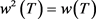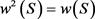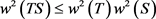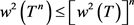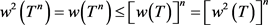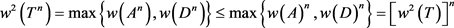NOTES

*通讯作者。

 吴德玉, 阿拉坦仓, 黄俊杰, 海国君. Hilbert空间中线性算子数值域及其应用[M]. 北京: 科学出版社, 2018.

 Markus, A., Matsaev, V. and Tretter, C. (2001) A New Concept for Block Operator Matrices: The Quadratic Numerical Range. Linear Algebra and Its Applications, 330, 89-112.
https://doi.org/10.1016/S0024-3795(01)00230-0

 Tretter, C. (2008) Spectral Theory of Block Operator Ma-trices and Applications. Imperial College Press, Covent Garden, London, UK.
https://doi.org/10.1142/p493

 Raodkm, G.K. (1997) Numerical Range. Springer-Verlag, New York.
https://doi.org/10.1007/978-1-4613-8498-4

 Sinclair, A.M. (1970) Eigenvalues in the Boundary of the Nu-merical Range. Pacific Journal of Mathematics, 35, 231-234.
https://doi.org/10.2140/pjm.1970.35.231

 Deyu, W. and Atatancang, C. (2011) Invertibility of Nonnegative Hamiltonian Operator with Unbounded Entries. rossref.org/SimpleTextQuery, 373, 410-413.
https://doi.org/10.1016/j.jmaa.2010.07.042

 Deyu, W. and Ata-tancang, C. (2011) Spectral Inclusion Properties of the Numerical Range in a Space with an Indeﬁnite Metric. Linear Algebra and Its Applications, 435, 1131-1136.
https://doi.org/10.1016/j.laa.2011.02.053

 吴德玉, 阿拉坦仓. 分块算子矩阵谱理论及其应用[M]. 北京: 科学出版社, 2013.

Top